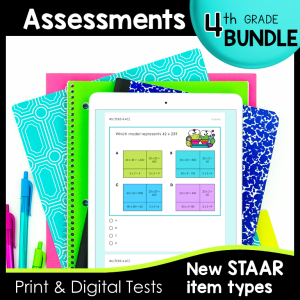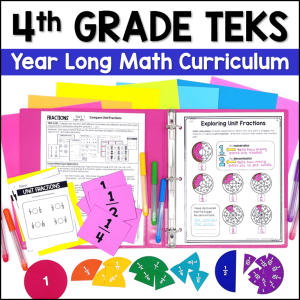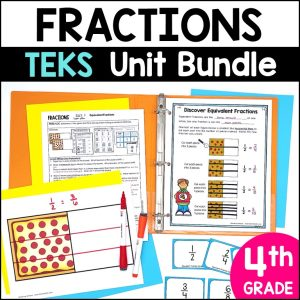Save 10% on your first order with code EXTRA10

# 4th Grade Fractions Tests – Digital and Print

\$4.00\$16.00187.25\$25.00
SKU: 3867222 Categories: ,

## Description

Need a 4th Grade STAAR Math Review that students can practice on a computer to master Fractions? If Test Prep wasn’t already hard enough, now students need to learn how to solve problems on a screen and get used to the new STAAR item types. No worries, we have you covered with these TEKS-aligned Printable and Digital Fraction Tests.

These tests were created with the new STAAR test questions guidelines in mind, and include drop down, multi-select, free response and multiple choice questions.

Fraction Topics:

• Represent Fractions as the Sum of Unit Fractions
• Decompose Fractions
• Determine if two given Fractions are Equivalent using a Variety of Methods
• Compare Fractions
• Add and Subtract Fractions with Like Denominators using Models
• Use Benchmark Fractions to Estimate Sums and Differences
• Represent Fractions and Decimals as Distances from Zero on a Number Line
• Relate Decimals to Fractions that name Tenths and Hundredths

What’s Included?

• 2 TEKS aligned Tests (Each Test is 10 questions)
• Printable versions of each Test
• Color Coded Teacher Keys with TEKS identified for each Question with room to create your small groups for reteach right on the key!

Great ways to use this resource:

• Unit Tests
• Easy-to-Assign Digital Activities
• No Prep Math Stations
• STAAR Review

The digital versions of the tests can be assigned via Google Classroom or any platform that supports Google Forms. The questions are STAAR formatted and include the NEW Problem Types. The assessments are in 2 formats: printable PDF and digital, self-grading Google Forms.

The assessments are created to give you meaningful feedback on student progress. There are more questions covering the Readiness Standards than the Supporting Standards, as the Readiness Standards are identified as the most critical content for success in subsequent grade levels. Readiness Standards also comprise 2/3 of the STAAR test.

Each set automatically grades itself when students click submit! I have identified the standard for every single problem in this bundle, to make data tracking super simple.

1. Easily Differentiate by making a separate copy of the Google Form and eliminating questions or answer choices. This is great way to adjust this resource to align with IEPs or the needs of your students.
2. Create multiple reviews from this resource, splitting up the questions to use in a variety of ways. Please note that the questions are not editable.

You do not need Google Classroom to use the digital form of this resource, but you must have a Google e-mail to use Google Forms.

Fraction TEKS Alignment:

4.3A Represent a fraction a/b as a sum of fractions 1/b, where a and b are whole numbers and b > 0, including when a > b.

4.3B Decompose a fraction in more than one way into a sum of fractions with the same denominator using concrete and pictorial models and recording results with symbolic representations.

4.3C Determine if two given Fractions are Equivalent using a Variety of Methods.

4.3D Compare two fractions with different numerators and different denominators and represent the comparison using the symbols >, =, or <.

4.3E Represent and solve addition and subtraction of fractions with equal denominators using objects and pictorial models that build to the number line and properties of operations.

4.3F Evaluate the reasonableness of sums and differences of fractions using benchmark fractions 0, 1/4, 1/2, 3/4, and 1, referring to the same whole.

4.3G Represent fractions and decimals to the tenths or hundredths as distances from zero on a number line.

4.2G Relate decimals to fractions that name tenths and hundredths.